## Introduction

Graphs are a set of objects where some pairs of objects called nodes or verticies are connected by links called edges. The nodes here can be seen numbered from 1 to 6. There are edges connecting these various nodes.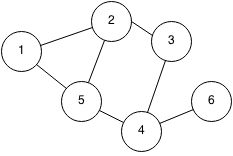A undirected graph is a graph where an edge from A to B is the same as the edge from B to A for all edges. The above graph is undirected.

A directed or bidirectional graph is a graph where edges have direction meaning if there is an edge from A to B then there may not be an edge from B to A.A subgraph is a subset of edges and vertices within a graph.

A directed acyclic graph (DAG) is a graph with no directed cycles (see topological sorting).

A weighted graph is a graph that contains weights or values assigned to each edge or node. Usually these weights act as the cost to reach/use that node.

## Graph Representations

A graph can be represented in various ways, but the most useful representations are as an adjacency matrix or as an adjacency list.

An adjacency matrix stores a graph of N nodes with a two dimensional NxN array. We let adjMatrix[i][j] represents the weight between the node i and node j. If we have a sparse graph which has many nodes but few edges, the memory storage will be very inefficient and it would be better to use an adjacency list. However, if the graph is very dense, or there are few nodes, then an adjacency matrix is quick and easy to use. Checking if an edge exists between two nodes is O(1).

Example:The adjacency matrix of the graph is:

1 2 3 4 5 6
1 0 1 0 0 1 0
2 1 0 1 0 1 0
3 0 1 0 1 0 0
4 0 0 1 0 1 1
5 1 1 0 1 0 0
6 0 0 0 1 0 0

An adjacency list stores a graph in an array of linked lists. Each node stores its neighbours by keeping a linked list of edges. Adjacency lists are slower when checking if an edge exists but they are more memory efficient since they only need to store the total number of edges.

Example:The adjacency list of the graph is:

Node edges
1 2 5
2 1 3 5
3 2 4
4 3 5 6
5 1 2 4
6 4

## Tree

A tree is a special graph with no cycles. It has the special property that there will be only one path from one node to another node.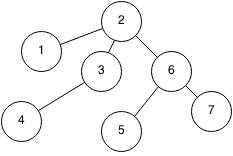A subtree is a child tree of a tree.

Note that trees have two meanings in computer science. It can either refer to a tree data structure or it can refer to a tree in graph theory.

### Spanning Tree

A spanning tree of a graph is a connected tree that spans all the nodes of the graph.

A minimum spanning tree is the spanning tree that requires the minimum of some property such as total weight or total edges.

Spanning tree algorithms are essential in networking to ensure no loops occur when sending data through a network.

Algorithm Desc Time Space
Prim's Using greedy method O(n log n) O(n2)
Kruskal Using connected components O(n log n) O(n2)

## Shortest Path

The shortest path is defined as a path from one node to another while trying to minimize a certain property (least number of nodes, smallest total weight). However, shortest paths may have negative weights which leads to cycles.

### Algorithms

Algorithm Desc Time Space Detect cycles?
Floyd Warshall Computes shortest path between all pairs of nodes O(n3) O(n2) Yes
Bellman Ford Computes shortest path between a pairs of nodes O(n2) O(n) Yes
Dijkstra's Computes shortest path between a pair of nodes using the Greedy method O(n log n) O(n log n) No

## Cycle detection

A cycle occurs when you start at a node and you can reach the same node from a path.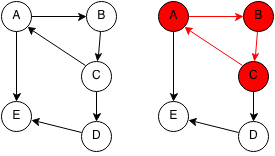Note that trees cannot contain cycles.

A cycle can be detected using a depth first search on each unvisited node to check if the DFS tree has a backwards edge.

## Topological Sorting

A topological sort or topological order of a directed graph is an order in which every node will come after its ancestors.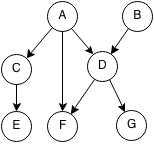For example topological orders could be:

• (A, B, C, D, E, F G)
• (B, A, D, C, F, E, G)

But (B, A, C, F, D, E, G) is not a topological ordering because D is an ancestor of F and it comes after F.

## Strongly Connected

A graph is strongly connected if all nodes in the graph follows the conditions:

• There exists a path from that node to every other node
• All other nodes can visit that node.

In other words, all nodes in a strongly connected graph can visit each other.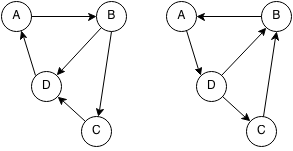To determine if a graph is strongly connected, we first pick a node and check that we can reach all nodes from it and this checks the first property. To check the second property, we can reverse all the edges of a node (A->B becomes B->A) and then check again that we can reach all nodes from the same node.

## Connected Components

A connected component is a subgraph where all the vertices in the subgraph connect to each other. Finding the number of distinct connected components can be done using a breadth first search or a depth first search.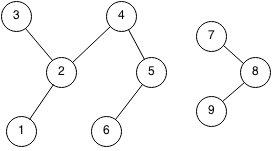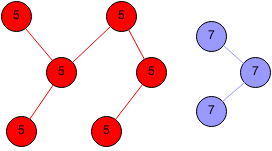### Strongly Connected Components

A strongly connected component is a connected component but has the property that each vertex can visit any other vertex in the strongly connected component from any path.